Electron. J. Diff. Eqns., Vol. 2003(2003), No. 64, pp. 1-8.

### Self-adjointness of Schrodinger-type operators with singular potentials on manifolds of bounded geometry Ognjen Milatovic

Abstract:
We consider the Schrodinger type differential expression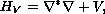where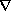is a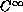-bounded Hermitian connection on a Hermitian vector bundle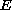of bounded geometry over a manifold of bounded geometry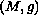with metricand positive-bounded measure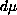, and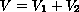, where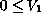in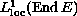and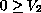inare linear self-adjoint bundle endomorphisms. We give a sufficient condition for self-adjointness of the operator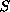in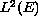defined by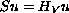for all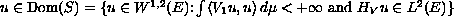. The proof follows the scheme of Kato, but it requires the use of more general version of Kato's inequality for Bochner Laplacian operator as well as a result on the positivity of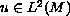satisfying the equation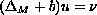, where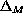is the scalar Laplacian on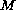,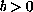is a constant and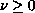is a positive distribution on.

Submitted May 13, 2003. Published June 11, 2003.
Math Subject Classifications: 35P05, 58J50, 47B25, 81Q10.
Key Words: Schrodinger operator, self-adjointness, manifold, bounded geometry, singular potential

Show me the PDF file (209K), TEX file, and other files for this article.Ognjen Milatovic 78 Apsley Street, Apt. 1 Hudson, MA 01749, USA email: omilatov@unf.edu